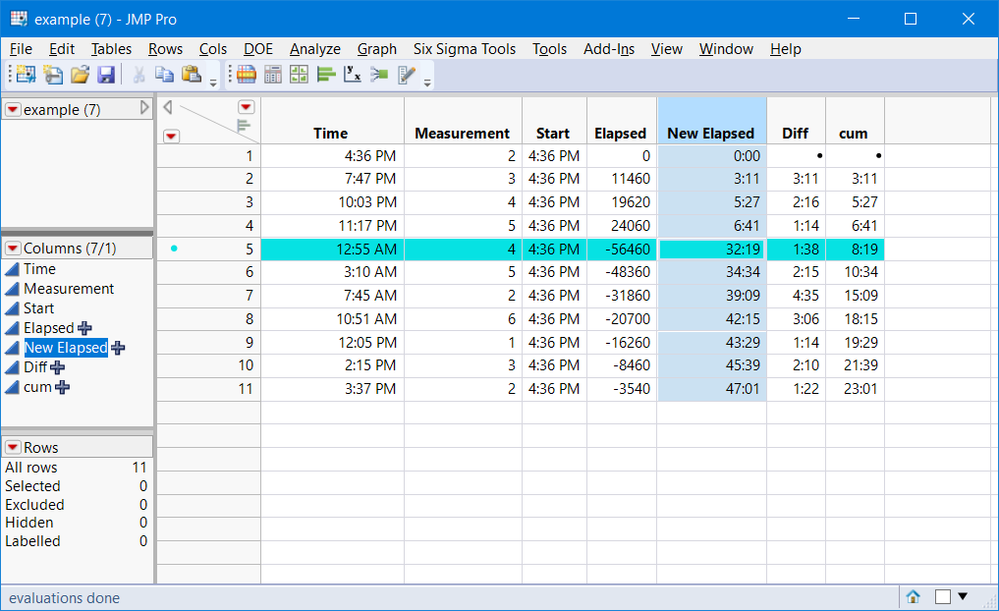Choose Language Hide Translation Bar
Highlighted

## Working with hh:mm columns that pass through midnight to determine elapsed times.

I thought this was going to be a straightforward problem, and here I am, a few hours later no further ahead. I have a two columns in very large table. One is a time in format hh:mm. The other is a series of measurements. All the measurements are taken over a 24 hour period  (or slightly less) and the time recorded as hh:mm. What I really need is an elapsed time from the first measurement. The problem is that everything is referenced to 00:00 and so the calculations go all whacky at that point as it doesn't know it is the next day and the data set is far too big for me to add the day manually. Any and all thoughts are gratefully appreciated!

The other weird thing I found was that the data file behaved quite differently depending upon whether I imported the Excel file, or save the Excel file as a .txt file and imported it. The latter was much better.

9 REPLIES 9
Highlighted

## Re: Working with hh:mm columns that pass through midnight to determine elapsed times.

1. Is the time column a character column, or a JMP Time column?
2. Would the detection of the current rows time value being less than the previous rows value be an indicator of an increment in the date?
Jim
Highlighted

## Re: Working with hh:mm columns that pass through midnight to determine elapsed times.

@txnelson  Thanks very much.

The column is a JMP numerical continuous formatted in JMP hh:mm format. I also converted it to character to see if that helped (it didn't).

I've tried sorting the column such that it begins at eg. 00:00. And that works. But unfortunately a measurement at 16:00 (day 1) will be right next to 16:10 (taken on day 2).

I did try the Lag command in the formula editor which was looking good but again as soon as you crossed that 00:00 threshold is got kind of crazy. Then I started building some if/then statements in formula which I will return to as I was getting warm there. But I didn't think of using the if/then statements to toggle the data somehow as you suggest. And by then it was 3 am and thought it was time to head to bed.

Thanks very much for your thoughts.

Best,

Greg

Highlighted

## Re: Working with hh:mm columns that pass through midnight to determine elapsed times.

I just made a sample table that hopefully shows the problem better than my description.

Highlighted

## Re: Working with hh:mm columns that pass through midnight to determine elapsed times.

Here is the best I can do, without an exact Day value.  The JSL below makes the assumption that a new day has started if the previous row value for the column Time, is less than the current value of the column Time.  You indicated in a previous response that this may not be the case.  But, without an exact Day value, this is the best that I think that can be done.  I have also attached your data table with the new formula column attached.  Here is the proposed formula

``````If( Row() == 1,
dayCounter = 0;
startTime = :Time;
newElapsedtime = 0;
,
If( :Time < Lag( :Time ),
dayCounter
++);
If( dayCounter == 0,
newElapsedtime = :Time - startTime,
newElapsedtime = (In Days( 1 ) - startTime) + In Days( dayCounter ) + :Time
);
);
newElapsedtime;``````
Jim
Highlighted

## Re: Working with hh:mm columns that pass through midnight to determine elapsed times.

Thanks again @txnelson . Much appreciated!

Highlighted

## Re: Working with hh:mm columns that pass through midnight to determine elapsed times.

I looked for an alternate solution, because I thought your function in a large table might be slow.  My approach is to use dif and lag functions to create column :cum cumulative time. In otherwords get incremental time changes then sum them up.

I think there is an error in your function, but I am not sure where. I converted the table to duration format.Here is the formula for  :dif

``````If( :Time < Lag( :Time, 1 ),
(:Time + 24 * 60 * 60) - Lag( :Time, 1 ),
Dif( :Time, 1 )
)``````

Here is the formula for :cum

``Sum(Lag(:cum, 1), :Diff)``

This assumes there is less than 24 hrs between events.

Jim, I think you need In Days(dayCounter -1) in your formula, since you counted 1 day for your starttime.

``````If( Row() == 1,
dayCounter = 0;
startTime = :Time;
newElapsedtime = 0;
,
If( :Time < Lag( :Time ),
dayCounter
++);
If( dayCounter == 0,
newElapsedtime = :Time - startTime,
newElapsedtime = (In Days( 1 ) - startTime) + In Days( dayCounter - 1 ) +
:Time
);
)``````
Highlighted

Good catch.

Jim
Highlighted

## Re: Working with hh:mm columns that pass through midnight to determine elapsed times.

This might be unnecessary, but it was fun so and I got to use parallel assign().

``````Names default to here(1);
dt = current data table();
t = dt:Time << Get Values();
n = nitems(t);
diff = t[2::n]-t[1::(n-1)];
day_break = loc(diff<0); // this will give you last row of the day

// make a matrix n x number of day breaks
m = J(n, nitems(day_break), -1);
parallel assign({db = day_break},
// make the item a 1 if it's row is higher than the corresponding row
m[a, b] = a > db[b];
);
m;
// now just sum the matrix
day = VSum(m`); // +1; // if you want the day to start at 1
dt << New Column("Day", ordinal, <<Set Values(day));
``````
Vince Faller - Predictum
Highlighted

## Re: Working with hh:mm columns that pass through midnight to determine elapsed times.

cool

Jim
Article Labels

There are no labels assigned to this post.